Data-driven businesses use technology as an insight platform to empower nontechnical users.

The post Data-driven to business insights appeared first on The Data Roundtable.“Analytics Can Save Higher Education. Really.” is a call to action for the higher education community to leverage data and analytics for better decision making at colleges and universities. It stresses the importance of using data and analytics to improve student outcomes, campus operations and much more. Oklahoma State University [...]In response to a recent article about how to compute the cosine similarity of observations, a reader asked whether it is practical (or even possible) to perform these types of computations on data sets that have many thousands of observations. The problem is that the cosine similarity matrix is an N x N matrix, where N is the sample size. Thus, the matrix can be very big even for moderately sized data.

The same problem exists for all distance- and similarity-based measurements. Because the Euclidean distance is familiar to most readers, I will use distance to illustrate the techniques in this article. To be specific, suppose you have N=50,000 observations. The full distance matrix contains 2.5 billion distances between pairs of observations. However, in many applications, you are not interested in all the distances. Rather, you are interested in extreme distances, such as finding observations that are very close to each other or are very far from each other. For definiteness, suppose you want to find observations that are less than a certain distance from each other. That distance (called the cutoff distance) serves as a filter. Instead of storing N2 observations, you only need to store the observations that satisfy the cutoff criterion.

### Compute by using block-matrix computations

A way to perform a distance computation is to divide the data into blocks and perform block-matrix computations. If X is the data matrix, then the pairwise distance matrix of the N rows is an N x N matrix. But you can subdivide X into blocks where each block has approximately M rows, where M is a smaller number such as M=1,000. Write X = [A1, A2, ..., Ak], where the block matrices are stacked vertically. Then the full distance matrix D = dist(X, X) is equal to stacking the distance matrices for each of the block matrices: D = [dist(A1,X), dist(A2,X), ..., dist(Ak,X)]. Each of the block matrices is an M x N matrix. The following diagram shows the block-matrices. The main idea is to compute each block matrix (which fits into memory), then identify and extract the values that satisfy the cutoff distance. With this technique, you never hold the full N x N distance matrix in memory.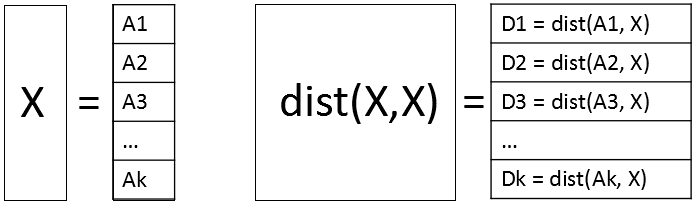### A small example of block computations

A programming principle that I strongly endorse is "start small." Even if your goal is to work with large data, develop and debug the program for small data. In this section, the data contains only 13 observations and 10 variables. The goal is to use block-matrix computations (with a block size of M=3) to compute the 132 = 169 pairwise distances between the observations. As each block of distances is computed, those distances that are less than 2 (the cutoff distance in this example) are identified and stored. The data are simulated by using the following DATA step:

```/* start with a smaller example: 13 observations and 10 vars */ %let N = 13; data Have; call streaminit(12345); array x; do i = 1 to &N; do j = 1 to dim(x); x[j] = rand("Uniform", -1, 1); end; output; end; keep x:; run;```

It makes sense to report the results by giving the pair of observations numbers and the distance for those pairs that satisfy the criterion. For these simulated data, there are 10 pairs of observations that are closer than 2 units apart, as we shall see shortly.

The following SAS/IML program shows how to use block computation to compute the pairs of observations that are close to each other. For illustration, set the block size to M=3. Suppose you are computing the second block, which contains the observations 4, 5, and 6. The following program computes the distance matrix from those observations to every other observation:

```proc iml; use Have; read all var _NUM_ into X; close; m = 3; /* block size */ n = nrow(X); /* number of observations */ cutoff = 2; /* report pairs where distance < cutoff */   /* Block matrix X = [A1, A2, A3, ..., Ak] */ startrow=4; endrow=6; /* Example: Look at 2nd block with obs 4,5,6 */ rows = startRow:endRow;   /* Compute D_i = distance(Ai, X) */ A = X[rows,]; /* extract block */ D = distance(A, X); /* compute all distances for obs in block */ print D[r=rows c=(1:n) F=5.2];```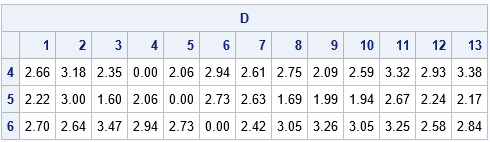The output shows the distances between the 4th, 5th, and 6th observations and all other observation. From the output, you can see that

• The 4th observation is not less than 2 units from any observation (except itself).
• The 5th observation is within 2 units of observations 3, 8, 9, and 10.
• The 6th observation is not less than 2 units from any observation.

The following statements use the LOC function to locate the elements of D that satisfy the cutoff criterion. (You should always check that the vector returned by the LOC function is nonempty before you use it.) The NDX2SUBS function converts indices into (row, col) subscripts. The subscripts are for the smaller block matrix, so you have to map them to the row numbers in the full distance matrix:

```/* Extract elements that satisfy the criterion */ idx = loc( D < cutoff ); /* find elements that satisfy cutoff criterion */ *if ncol(idx) > 0 then do; /* should check that idx is not empty */ Distance = D[idx]; /* extract close distances */ s = ndx2sub(dimension(D), idx); /* s contains matrix subscripts */ Row = rows[s[,1]]; /* convert rows to row numbers for full matrix */ Col = s[,2]; print Row Col Distance[F=5.2];```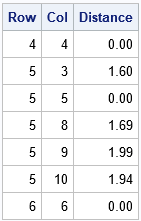Because the distance matrix is symmetric (and we can exclude the diagonal elements, which are 0), let's agree to report only the upper-triangular portion of the (full) distance matrix. Again, you can use the LOC function to find the (Row, Col) pairs for which Row is strictly less than Col. Those are the pairs of observations that we want to store:

``` /* filter: output only upper triangular when full matrix is symmetric */ k = loc( Row < Col ); /* store only upper triangular in full */ *if ncol(k)>0 then do; /* should check that k is not empty */ Row = Row[k]; Col = Col[k]; Distance = Distance[k]; print Row Col Distance[F=5.2];```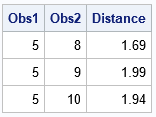Success! The printed observations are the elements of the upper-triangular distance matrix that satisfy the cutoff criterion. If you repeat these computations for other blocks (observations 1-3, 4-6, 7-9, 10-12, and 13) then you can compute all pairs of observations that are less than 2 units from each other. For the record, here are the 10 pairs of observations that are within 0.5 units of each other. Although the point of this article is to avoid computing the full 13 x 13 distance matrix, for this small example you can check the following table by visual inspection of the full distance matrix, F = distance(X, X).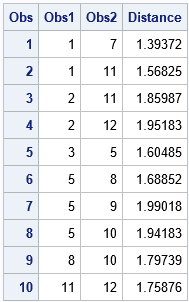### Block computations to compute distances in large data

The previous section describes the main ideas about using block matrix computations to perform distance computations for large data when the whole N x N distance matrix will not fit into memory. The same ideas apply to cosine similarity and or other pairwise computations. For various reasons, I recommend that the size of the block matrices be less than 2 GB.

The following DATA step generates 50,000 observations, which means there are 2.5 billion pairs of observations. The program computes all pairwise distances and finds all pairs that are within 0.5 units. The full distance matrix is about 18.6 GB, but the program never computes the full matrix. Instead, the program uses a block size of 5,000 rows and computes a total of 10 distance matrices, each of which requires 1.86 GB of RAM. (See "How much RAM do I need to store that matrix?) For each of these block matrices, the program finds and writes the pairs of observations that are closer than 0.5 units. The program requires about a minute to run on my desktop PC. If you have a slow PC, you might want to use N=20000 to run the example.

```/* Create 10 variables and a large number (N) of obs. The complete distance matrix requires N^2 elements. */ %let N = 50000; data Have; call streaminit(12345); array x; do i = 1 to &N; do j = 1 to dim(x); x[j] = rand("Uniform", -1, 1); end; output; end; keep x:; run;   /* Find pairs of observations that within 'cutoff' of each other. Write these to a data set. */ proc iml; use Have; read all var _NUM_ into X; close; n = nrow(X); /* number of observations */ m = 5000; /* block size: best if n*m elements fit into 2 GB RAM */ cutoff = 0.5; /* report pairs where distance < cutoff */   startRow = 1; /* set up output data set to store results */ create Results var {'Obs1' 'Obs2' 'Distance'}; do while (startRow <= n); /* Block matrix X = [A1, A2, A3, ..., Ak] */ endRow = min(startRow + m - 1, n); /* don't exceed size of matrix */ rows = startRow:endRow; /* Compute D_i = distance(Ai, X) */ A = X[rows,]; /* extract block */ D = distance(A, X); /* compute all distances for obs in block */   /* Extract elements that satisfy the criterion */ idx = loc( D < cutoff ); /* find elements that satisfy cutoff criterion */ if ncol(idx) > 0 then do; Distance = D[idx]; /* extract close distances */ s = ndx2sub(dimension(D), idx); /* s contains matrix subscripts */ Row = rows[s[,1]]; /* convert rows to row numbers for full matrix */ Col = s[,2]; /* filter: output only upper triangular when full matrix is symmetric */ k = loc( Row < Col ); /* store only upper triangular in full */ if ncol(k)>0 then do; Obs1 = Row[k]; Obs2 = Col[k]; Distance = Distance[k]; append; /* Write results to data set */ end; end; startRow = startRow + m; end; close; QUIT;   title "Distances Less than 0.5 for Pairs of Observations"; title2 "N = 50,000"; proc sgplot data=Results; histogram Distance; run;```

From the 2.5 billion distances between pairs of observations, the program found 1620 pairs that are closer than 0.5 units in a 10-dimensional Euclidean space. The distribution of those distances is shown in the following histogram.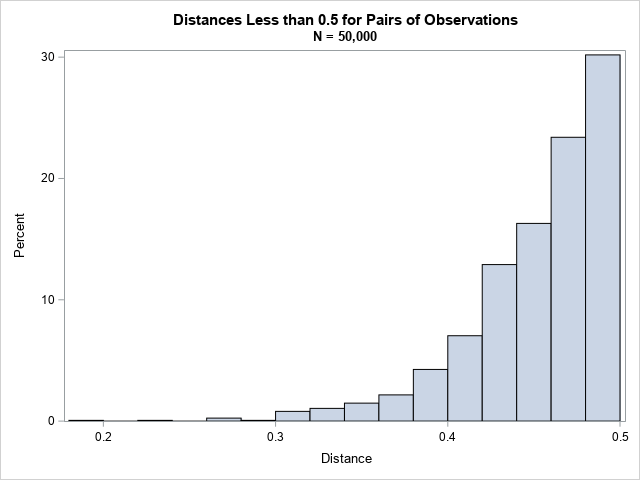### Further improvements

When the full matrix is symmetric, as in this example, you can improve the algorithm. Because only the upper triangular elements are relevant, you can improve the efficiency of the algorithm by skipping computations that are in the lower triangular portion of the distance matrix.

The k_th block matrix, A, represents consecutive rows in the matrix X. Let L be the row number of the first row of X that is contained in A. You do not need to compute the distance between rows of A and all rows of X. Instead, set Y = X[L:N, ] and compute distance(A, Y). With this modification, you need additional bookkeeping to map the column numbers in the block computation to the column numbers in the full matrix. However, for large data sets, you decrease the computational time by almost half. I leave this improvement as an exercise for the motivated reader.

### Conclusions

In summary, you can use a matrix langue like SAS/IML to find pairs of observations that are close to (or far from) each other. By using block-matrix computations, you never have to compute the full N x N distance matrix. Instead, you can compute smaller pieces of the matrix and find the pairs of observations that satisfy your search criterion.

Because this problem is quadratic in the number of observations, you can expect the program to take four times as long if you double the size of the input data. So the program in this article will take about four minutes for 100,000 observations and 16 minutes for 200,000 computations. If that seems long, remember that with 200,000 observations, the program must compute 40 billion pairwise distances.

The post Perform matrix computations when the matrices don't fit in memory appeared first on The DO Loop.## PROC SQL

PROC SQL is a very powerful ANSI 92 compliant version of SQL that also allows us to leverage many unique SAS capabilities. Recently I was asked if the PROC SQL in Figure 1 could be refactored into PROC FedSQL so it could run faster by leveraging SAS Viya’s in-memory engine CAS (SAS® Cloud Analytic Services). I was struggling to find a way to refactor this into PROC FedSQL, so I reached out to the SAS Jedi (aka Mark Jordan) for help.

```/* Original SQL Statements */ proc sql; create table BenchMark as select count(*) as ItemCount , sum( abs( nhits - nruns ) < 0.1*natbat ) as DIFF_10 from sashelp.baseball; run;```

Figure 1. Original PROC SQL

In Figure 2, we can review the SAS Log of our PROC SQL code.

• It is line 77 that we want to refactor into PROC FedSQL so we can benefit performance improvements by running that code distributed in CAS.
• On line 77, we use the alias DIFF_10 to create the new column name that is calculated by the two SAS functions SUM and ABS.
• The expression on line 77 will cause SQL to return a value of 1 if the condition is true and a value of 0 if the condition is false.
• The alias DIFF_10 will contain the summation of the value returned by the condition (i.e. 0 or 1) for all rows in our data set SASHELP.BASESBALL.

In Figure 5, we can review the results of our PROC SQL statement.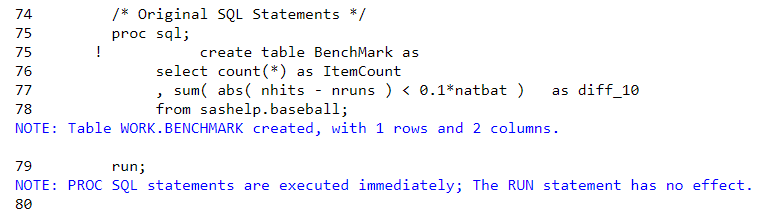Figure 2. SAS Log of PROC SQL

## PROC FedSQL

PROC FedSQL is ANSI 99 compliant without any of the unique SAS capabilities that PROC SQL contains, but PROC FedSQL is CAS enabled, which allows us to leverage SAS Viya’s distributed computing capabilities to improve run-times. Figure 3 is the refactored PROC FedSQL code that the SAS Jedi came up with.

```/* PROC FedSQL code */ proc fedsql sessref=casauto; create table BenchMark as select count(*) as ItemCount , sum(case when (abs (nhits - nruns ) < (0.1*natbat) ) is true then 1 end ) as DIFF_10 from baseball; quit;```

Figure 3. CAS-enabled PROC FedSQL

Figure 4 contains the SAS Log of our CAS enabled PROC FedSQL.

• Notice on lines 77 we added a CASE statement to the SUM function for our alias DIFF_10.
• On lines 78-79, the WHEN statement return a value of 1 when the condition is true and a value of 0 when it is false.
• The alias DIFF_10 will contain the summation, of the value returned by the CASE statement (i.e. 0 or 1) for all rows in our CAS table BASESBALL.

In Figure 5 we can review the results of our PROC FedSQL statement.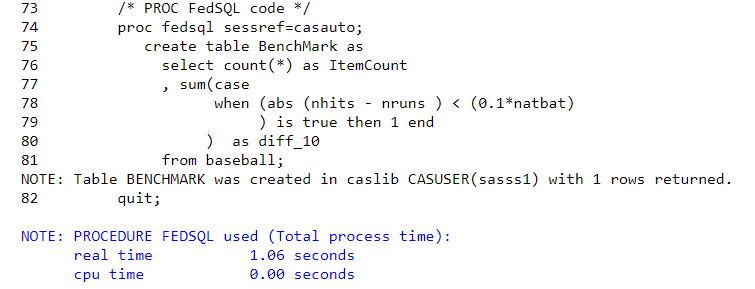Figure 4. SAS log of PROC FedSQL code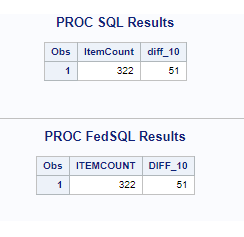Figure 5. Validation that the values from the refactoring of PROC SQL into PROC FedSQL match

## Conclusion

As we adopt SAS Viya, a goal is to leverage CAS to speed up the processing of routines written in PROC SQL. To accomplish this, refactor PROC SQL code into PROC FedSQL code. For PROC SQL that cannot be refactored, simply run that PROC SQL code as-is in SAS Viya’s SAS Programming Run-time Environment (SPRE).I am excited to announce that the sixth edition of The Little SAS Book is now available. We spent over a year rewriting and updating, and this may well be the best edition yet.

You can download a sample chapter or purchase e-book versions (PDF, EPUB or Kindle) by visiting SAS Press’ site.

If, like me, you like to be able to flip the pages and make notes in the margin, then you can get a hard copy (in paperback or hardback!) from Amazon.

When my younger son grabs a book or a toy from his older siblings without permission, his line of defense is always the same: “Sharing is caring!” Our kids' schools teach and reinforce this philosophy. Likewise, our family has rules to ensure peaceful, orderly sharing.

Similarly, many organizations value collaboration. They encourage researchers, data owners, data scientists and business analysts to share work product and ideas and facilitate it among their teams. As with families, they often find it easier said than done.

A big part of my job is to meet customers and advise them on how SAS technology can help solve their business challenges. A recurring topic has been around SAS Viya, the analytics capabilities collectively known as the SAS® Platform. I emphasize how SAS Viya seamlessly enables collaboration across diverse users and teams.

## SAS Viya collaboration use case with Commitments of Traders data

How does it work in real life? Here is an example to demonstrate how SAS coders and business analysts can easily collaborate on SAS Viya. I am using a publicly available data set known as Commitments of Traders (COT) that the U.S. Commodity Futures Trading Commission (CFTC) publishes on its website (https://www.cftc.gov/MarketReports/CommitmentsofTraders/index.htm.) Traders and researchers closely watch and analyze this data set for trends and price movements in the commodities market.

## A SAS programmer readies the data

First, I need to bring the original COT file, saved in ‘.txt’ format, to my enterprise SAS environment. On SAS Viya, I have a choice of using a programmatic or graphical user interface (GUI) approach to import data and perform data wrangling/preparation. Both interfaces are easily accessible from a drop-down menu on SAS Drive, a web-based central hub for SAS Viya applications (see Figure 1). I choose the programmatic approach by clicking on Develop SAS Code from the drop-down menu.

SAS® Studio, the programming interface for SAS Viya, is a web-based development environment that includes code autocomplete, a library of frequently used code snippets, pre-built GUI wizards for numerous analytical routines, etc.

In SAS Studio, I prepare the data with five steps: 1) Import the raw text file; 2) Reduce the number of variables; 3) Compute the traders’ net position; 4) Import the mapping table; and 5) Add the commodity category variable from the mapping table. For all of these steps, I use SAS DATA Step and PROC statements that have been around for 40+ years – old school SAS.

The last two steps in my program, Step 6 and Step 7, are unique to SAS Viya (see Figure 2 below.) Those lines of code start the Cloud Analytics Server (CAS) session and load the final curated table into CAS, an in-memory distributed analytical engine that I consider the “heart and soul” of SAS Viya. Once the data is in CAS, an authorized user of my enterprise SAS Viya environment can easily locate data in CAS library/catalog for data exploration, modeling, or developing business intelligence content.

## A business analyst creates and shares an interactive dashboard

Now it's the business analyst's turn. In this example, my teammate wants to build an interactive dashboard using curated COT data that I (the programmer) loaded into CAS. The analyst will access SAS Drive, select Explore and Visualize Data option from the menu, and be directed to SAS Visual Analytics, a web-based application that allows you to explore data and build point-and-click, interactive visualizations, no coding skills required.

Figure 3 and Figure 4 below show examples of the types of drillable dashboards and reports you can easily develop in SAS Visual Analytics with a few clicks. You can share the report internally via web link, view it in MS Office products, or publish it on the intranet or external facing website.

## Collaboration bridges diverse skill sets, fosters successful projects

Studies show that success of any analytical project requires multi-disciplinary teams that include database administrators, data scientists, analysts, subject matter experts, management and IT support. SAS Viya helps them capitalize on their strengths to promote frictionless collaboration in a secure and controlled environment.

This post, focused on collaboration between SAS coders and business analysts, only scratches the surface of SAS Viya's collaboration and knowledge sharing capabilities. Likewise, open source coders (R and Python) and business analysts can collaborate on SAS Viya too.

Free trials below give programmers and business analysts a taste of what's possible with SAS Viya. Try one and tell us about it in the comments.

How SAS® Viya fosters collaboration was published on SAS Users.When my younger son grabs a book or a toy from his older siblings without permission, his line of defense is always the same: “Sharing is caring!” Our kids' schools teach and reinforce this philosophy. Likewise, our family has rules to ensure peaceful, orderly sharing.

Similarly, many organizations value collaboration. They encourage researchers, data owners, data scientists and business analysts to share work product and ideas and facilitate it among their teams. As with families, they often find it easier said than done.

A big part of my job is to meet customers and advise them on how SAS technology can help solve their business challenges. A recurring topic has been around SAS Viya, the analytics capabilities collectively known as the SAS® Platform. I emphasize how SAS Viya seamlessly enables collaboration across diverse users and teams.

## SAS Viya collaboration use case with Commitments of Traders data

How does it work in real life? Here is an example to demonstrate how SAS coders and business analysts can easily collaborate on SAS Viya. I am using a publicly available data set known as Commitments of Traders (COT) that the U.S. Commodity Futures Trading Commission (CFTC) publishes on its website (https://www.cftc.gov/MarketReports/CommitmentsofTraders/index.htm.) Traders and researchers closely watch and analyze this data set for trends and price movements in the commodities market.

## A SAS programmer readies the data

First, I need to bring the original COT file, saved in ‘.txt’ format, to my enterprise SAS environment. On SAS Viya, I have a choice of using a programmatic or graphical user interface (GUI) approach to import data and perform data wrangling/preparation. Both interfaces are easily accessible from a drop-down menu on SAS Drive, a web-based central hub for SAS Viya applications (see Figure 1). I choose the programmatic approach by clicking on Develop SAS Code from the drop-down menu.

SAS® Studio, the programming interface for SAS Viya, is a web-based development environment that includes code autocomplete, a library of frequently used code snippets, pre-built GUI wizards for numerous analytical routines, etc.

In SAS Studio, I prepare the data with five steps: 1) Import the raw text file; 2) Reduce the number of variables; 3) Compute the traders’ net position; 4) Import the mapping table; and 5) Add the commodity category variable from the mapping table. For all of these steps, I use SAS DATA Step and PROC statements that have been around for 40+ years – old school SAS.

The last two steps in my program, Step 6 and Step 7, are unique to SAS Viya (see Figure 2 below.) Those lines of code start the Cloud Analytics Server (CAS) session and load the final curated table into CAS, an in-memory distributed analytical engine that I consider the “heart and soul” of SAS Viya. Once the data is in CAS, an authorized user of my enterprise SAS Viya environment can easily locate data in CAS library/catalog for data exploration, modeling, or developing business intelligence content.

## A business analyst creates and shares an interactive dashboard

Now it's the business analyst's turn. In this example, my teammate wants to build an interactive dashboard using curated COT data that I (the programmer) loaded into CAS. The analyst will access SAS Drive, select Explore and Visualize Data option from the menu, and be directed to SAS Visual Analytics, a web-based application that allows you to explore data and build point-and-click, interactive visualizations, no coding skills required.

Figure 3 and Figure 4 below show examples of the types of drillable dashboards and reports you can easily develop in SAS Visual Analytics with a few clicks. You can share the report internally via web link, view it in MS Office products, or publish it on the intranet or external facing website.

## Collaboration bridges diverse skill sets, fosters successful projects

Studies show that success of any analytical project requires multi-disciplinary teams that include database administrators, data scientists, analysts, subject matter experts, management and IT support. SAS Viya helps them capitalize on their strengths to promote frictionless collaboration in a secure and controlled environment.

This post, focused on collaboration between SAS coders and business analysts, only scratches the surface of SAS Viya's collaboration and knowledge sharing capabilities. Likewise, open source coders (R and Python) and business analysts can collaborate on SAS Viya too.

Free trials below give programmers and business analysts a taste of what's possible with SAS Viya. Try one and tell us about it in the comments.

How SAS® Viya fosters collaboration was published on SAS Users.Computing rates and proportions is a common task in data analysis. When you are computing several proportions, it is helpful to visualize how the rates vary among subgroups of the population. Examples of proportions that depend on subgroups include:

• Mortality rates for various types of cancers
• Incarceration rates by race

### Uncertainty in estimates

An important fact to remember is that the uncertainty in an estimate depends on the sample size. If a small college has 8 physics majors and 5 of them graduate in four years, the graduation rate in physics is 0.6. However, because of the small sample size, the uncertainty in that estimate is much greater than for a larger group, such as if the English department graduates 50 out of 80 students. Specifically, if the estimate of a binomial proportion is p, the standard error of the estimate is sqrt(p(1–p)/n), where n is the sample size. Thus for the physics students, the standard error is sqrt(0.6*0.4/8) = 0.17, whereas for the English majors, the standard error is sqrt(0.6*0.4/80) = 0.05.

Therefore, it is a good idea to incorporate some visual aspect of the uncertainty into any graph of proportions and rates. For analyses that involve dozens or hundreds of groups, you can use a funnel plot of proportions, which I have used to analyze adoption rates for children and immunization rates for kindergartens in North Carolina. When you have a smaller number of groups, a simple alternative is a dot plot with error bars that indicate either the standard error or a 95% confidence interval for the estimate. As I've explained, I prefer to display the confidence interval.

The Chronicle of Higher Education web site enables you to find the graduation rates for US colleges and universities. You can find the average graduation rate by states (50 groups) or by college (hundreds of groups). You can also find the graduation rate by race (five groups) for any individual college. Because most colleges have fewer Hispanic, Asian, and Native American students, it is important to indicate the sample size or the uncertainty in the empirical estimates.

I don't want to embarrass any small college, so the following data are fake but are typical of the group sizes that you might see in real data. Suppose a college has six majors, labeled as A, B, C, D, E, and F. The following SAS DATA step defines the number of students who graduated in four years (Grads) and the number of students in each cohort (Total).

```data Grads; input Major \$ Grads Total @@; datalines; A 10 22 B 10 32 C 17 25 D 4 7 E 8 14 F 16 28 ;```

### Manual computations of confidence intervals for proportions

If you use a simple Wald confidence interval, it is easy to write a short DATA step to compute the empirical proportions and a 95% confidence interval for each major:

```data GradRate; set Grads; GradRate = Grads / Total; p = Grads / Total; /* empirical proportion */ StdErr = sqrt(p*(1-p)/Total); /* standard error */ /* use Wald 95% CIs */ z = quantile("normal", 1-0.05/2); LCL = max(0, p - z*StdErr); /* LCL can't be less than 0 */ UCL = min(1, p + z*StdErr); /* UCL can't be less than 0 */ label p = "Proportion" LCL="Lower 95% CL" UCL="Upper 95% CL"; run;   proc print data=GradRate noobs label; var Major Grads Total p LCL UCL; run;```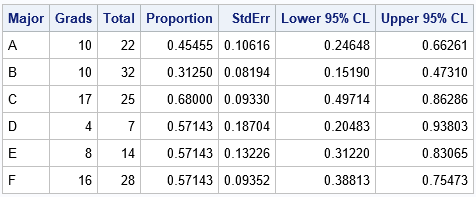The output shows that although majors D, E, and F have the same four-year graduation rate (57%), the estimate for the D group, which has only seven students, has twice as much variability as the estimate for the F group, which has four times as many students.

### Automating the computations by using PROC FREQ

Although it is easy enough to write a DATA step for the Wald CI, other types of confidence intervals are more complicated. The BINOMIAL option in the TABLES statement of PROC FREQ enables you to compute many different confidence intervals (CIs), including the Wald CI. In order to use these data in PROC FREQ, you need to convert the data from Event-Trials format to Event-Nonevent format. For each major, let Graduated="Yes" indicate the count of students who graduated in four years and let Graduated="No" indicate the count of the remaining students. The following data step converts the data and estimates the binomial proportion for each group:

```/* convert data to Event/Nonevent format */ data GradFreq; set Grads; Graduated = "Yes"; Count = Grads; output; Graduated = "No "; Count = Total-Grads; output; run;   /* Use PROC FREQ to analyze each group separately and compute the binomial CIs */ proc freq data=GradFreq noprint; by notsorted Major; tables Graduated / binomial(level='Yes' CL=wald); /* choose from among many confidence intervals */ weight Count; output out=FreqOut binomial; run;   proc print data=FreqOut noobs label; var Major N _BIN_ L_BIN U_BIN ; label _BIN_ = "Proportion" L_BIN="Lower 95% CL" U_BIN="Upper 95% CL"; run;```

The output is not shown because the estimates and CIs from PROC FREQ are identical to the estimates from the "manual" calculations in the previous section. However, by using PROC FREQ you can easily compute more sophisticated confidence intervals.

### Visualizing binomial proportions

As indicated earlier, it is useful to plot the proportions and confidence intervals. When you plot several proportions on the same graph, I recommend that you sort the data in some way, such as by the estimated proportions. If there are two groups that have the same proportion, you can use the size of the group to break the tie.

It can also be helpful to draw a reference line for the overall rate, regardless of group membership. (You can get the overall proportion by repeating the previous call to PROC FREQ but without using the BY statement.) For these data, the overall proportion of students who graduate in four years is 65/128 = 0.5078. Lastly, I think it is a good idea to add a table that shows the number of students in each major. You can use the YAXISTABLE statement to add that information to the graph, as follows:

```/* sort by estimated proportion; break ties by using CI */ proc sort data=FreqOut; by _BIN_ N; run;   title "Graduation Rates by College Major"; proc sgplot data=FreqOut; label _BIN_ = "Proportion" N="Number of Students"; scatter y=Major x=_BIN_ / xerrorlower=L_BIN xerrorupper=U_BIN; yaxistable N / y=Major location=inside position=left valueattrs=(size=9); refline 0.5078 / axis=x labelloc=inside label="Overall"; yaxis discreteorder=data offsetmax=0.1; /* preserve order of categories */ xaxis grid values=(0 to 1 by 0.1) valueshint; run;```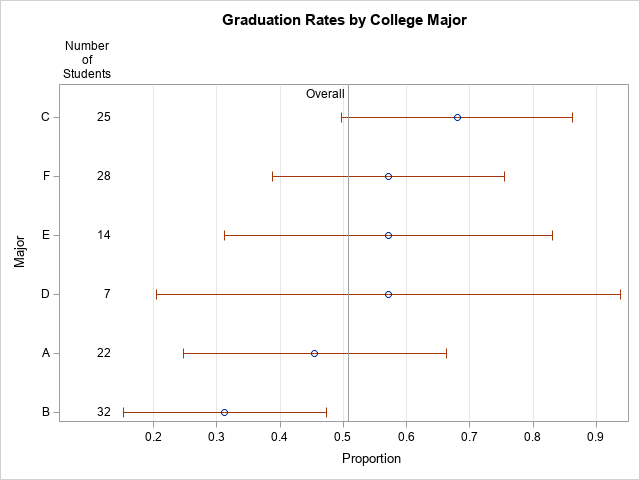The graph shows the range of graduation rates. The "error bars" are 95% CIs, which show that majors that have few students have larger uncertainty than majors that have more students. If there are 10 or more categories, I recommend that you use alternating color bands to make it easier for the reader to associate intervals with the majors.

In summary, this article shows how to use PROC FREQ to estimate proportions and confidence intervals for groups of binary data. A great way to convey the proportions to others is to graph the proportions and CIs. By including the sample size on the graph, readers can connect the uncertainty in the estimates to the sample size.

The post Compute and visualize binomial proportions in SAS appeared first on The DO Loop.Computing rates and proportions is a common task in data analysis. When you are computing several proportions, it is helpful to visualize how the rates vary among subgroups of the population. Examples of proportions that depend on subgroups include:

• Mortality rates for various types of cancers
• Incarceration rates by race

### Uncertainty in estimates

An important fact to remember is that the uncertainty in an estimate depends on the sample size. If a small college has 8 physics majors and 5 of them graduate in four years, the graduation rate in physics is 0.6. However, because of the small sample size, the uncertainty in that estimate is much greater than for a larger group, such as if the English department graduates 50 out of 80 students. Specifically, if the estimate of a binomial proportion is p, the standard error of the estimate is sqrt(p(1–p)/n), where n is the sample size. Thus for the physics students, the standard error is sqrt(0.6*0.4/8) = 0.17, whereas for the English majors, the standard error is sqrt(0.6*0.4/80) = 0.05.

Therefore, it is a good idea to incorporate some visual aspect of the uncertainty into any graph of proportions and rates. For analyses that involve dozens or hundreds of groups, you can use a funnel plot of proportions, which I have used to analyze adoption rates for children and immunization rates for kindergartens in North Carolina. When you have a smaller number of groups, a simple alternative is a dot plot with error bars that indicate either the standard error or a 95% confidence interval for the estimate. As I've explained, I prefer to display the confidence interval.

The Chronicle of Higher Education web site enables you to find the graduation rates for US colleges and universities. You can find the average graduation rate by states (50 groups) or by college (hundreds of groups). You can also find the graduation rate by race (five groups) for any individual college. Because most colleges have fewer Hispanic, Asian, and Native American students, it is important to indicate the sample size or the uncertainty in the empirical estimates.

I don't want to embarrass any small college, so the following data are fake but are typical of the group sizes that you might see in real data. Suppose a college has six majors, labeled as A, B, C, D, E, and F. The following SAS DATA step defines the number of students who graduated in four years (Grads) and the number of students in each cohort (Total).

```data Grads; input Major \$ Grads Total @@; datalines; A 10 22 B 10 32 C 17 25 D 4 7 E 8 14 F 16 28 ;```

### Manual computations of confidence intervals for proportions

If you use a simple Wald confidence interval, it is easy to write a short DATA step to compute the empirical proportions and a 95% confidence interval for each major:

```data GradRate; set Grads; GradRate = Grads / Total; p = Grads / Total; /* empirical proportion */ StdErr = sqrt(p*(1-p)/Total); /* standard error */ /* use Wald 95% CIs */ z = quantile("normal", 1-0.05/2); LCL = max(0, p - z*StdErr); /* LCL can't be less than 0 */ UCL = min(1, p + z*StdErr); /* UCL can't be less than 0 */ label p = "Proportion" LCL="Lower 95% CL" UCL="Upper 95% CL"; run;   proc print data=GradRate noobs label; var Major Grads Total p LCL UCL; run;```The output shows that although majors D, E, and F have the same four-year graduation rate (57%), the estimate for the D group, which has only seven students, has twice as much variability as the estimate for the F group, which has four times as many students.

### Automating the computations by using PROC FREQ

Although it is easy enough to write a DATA step for the Wald CI, other types of confidence intervals are more complicated. The BINOMIAL option in the TABLES statement of PROC FREQ enables you to compute many different confidence intervals (CIs), including the Wald CI. In order to use these data in PROC FREQ, you need to convert the data from Event-Trials format to Event-Nonevent format. For each major, let Graduated="Yes" indicate the count of students who graduated in four years and let Graduated="No" indicate the count of the remaining students. The following data step converts the data and estimates the binomial proportion for each group:

```/* convert data to Event/Nonevent format */ data GradFreq; set Grads; Graduated = "Yes"; Count = Grads; output; Graduated = "No "; Count = Total-Grads; output; run;   /* Use PROC FREQ to analyze each group separately and compute the binomial CIs */ proc freq data=GradFreq noprint; by notsorted Major; tables Graduated / binomial(level='Yes' CL=wald); /* choose from among many confidence intervals */ weight Count; output out=FreqOut binomial; run;   proc print data=FreqOut noobs label; var Major N _BIN_ L_BIN U_BIN ; label _BIN_ = "Proportion" L_BIN="Lower 95% CL" U_BIN="Upper 95% CL"; run;```

The output is not shown because the estimates and CIs from PROC FREQ are identical to the estimates from the "manual" calculations in the previous section. However, by using PROC FREQ you can easily compute more sophisticated confidence intervals.

### Visualizing binomial proportions

As indicated earlier, it is useful to plot the proportions and confidence intervals. When you plot several proportions on the same graph, I recommend that you sort the data in some way, such as by the estimated proportions. If there are two groups that have the same proportion, you can use the size of the group to break the tie.

It can also be helpful to draw a reference line for the overall rate, regardless of group membership. (You can get the overall proportion by repeating the previous call to PROC FREQ but without using the BY statement.) For these data, the overall proportion of students who graduate in four years is 65/128 = 0.5078. Lastly, I think it is a good idea to add a table that shows the number of students in each major. You can use the YAXISTABLE statement to add that information to the graph, as follows:

```/* sort by estimated proportion; break ties by using CI */ proc sort data=FreqOut; by _BIN_ N; run;   title "Graduation Rates by College Major"; proc sgplot data=FreqOut; label _BIN_ = "Proportion" N="Number of Students"; scatter y=Major x=_BIN_ / xerrorlower=L_BIN xerrorupper=U_BIN; yaxistable N / y=Major location=inside position=left valueattrs=(size=9); refline 0.5078 / axis=x labelloc=inside label="Overall"; yaxis discreteorder=data offsetmax=0.1; /* preserve order of categories */ xaxis grid values=(0 to 1 by 0.1) valueshint; run;```The graph shows the range of graduation rates. The "error bars" are 95% CIs, which show that majors that have few students have larger uncertainty than majors that have more students. If there are 10 or more categories, I recommend that you use alternating color bands to make it easier for the reader to associate intervals with the majors.

In summary, this article shows how to use PROC FREQ to estimate proportions and confidence intervals for groups of binary data. A great way to convey the proportions to others is to graph the proportions and CIs. By including the sample size on the graph, readers can connect the uncertainty in the estimates to the sample size.

The post Compute and visualize binomial proportions in SAS appeared first on The DO Loop.